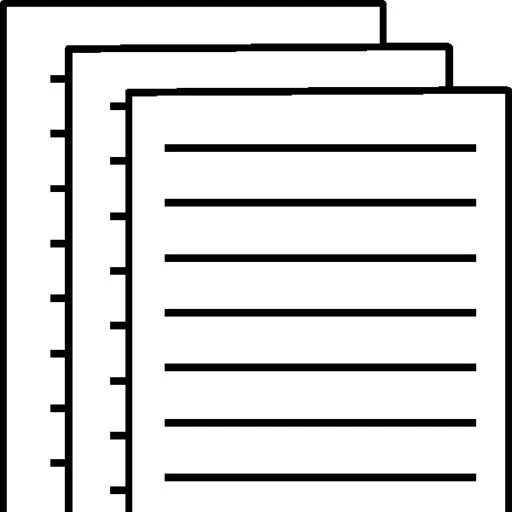## SBA 215 Project Week 7 solution

Using the ROI data set:

For each of the two majors:

Draw the scatter diagram of Y = ‘Annual % ROI’ against X = ‘Cost’.

The obtained two diagrams are given below,

Obtain b0 and b1 of the regression equation defined as y ̂ = b0 + b1X and the coefficient of determination (r2) from the Excel regression output.

For Business Major, the obtained output is given below.

SUMMARY OUTPUT Multiple R R Square Adjusted R Square Standard Error Observations 0.9701 0.9410 0.9377 0.0027 20 df SS MS F Significance F 1 0.0022 0.0022 287.2207 0.0000 18 0.0001 0.0000 19 0.0023 Coefficients Standard Error t Stat P-value Lower 95% Upper 95% 0.1180 0.0024 48.5862 0.0000 0.1129 0.1231 -2.11204E-07 1.24622E-08 -16.94758619 1.64456E-12 -2.37386E-07 -1.85022E-07

From the above output we can see that b0 = 0.1180 and b1= -2.11204E-07 thus the regression equation is,

Y = 0.1180-2.11204E-07*X

For Engineering Major, the obtained output is given below.

SUMMARY OUTPUT Multiple R R Square Adjusted R Square Standard Error Observations 0.9754 0.9515 0.9488 0.0033 20 df SS MS F Significance F 1 0.0039 0.0039 352.8738 0.0000 18 0.0002 0.0000 19 0.0041 Coefficients Standard Error t Stat P-value Lower 95% Upper 95% 0.1268 0.0020 62.7372 0.0000 0.1225 0.1310 -2.1455E-07 1.14214E-08 -18.78493483 2.83396E-13 -2.38545E-07 -1.90554E-07

From the above output we can see that b0 = 0.1268 and b1= -2.1455E-07 thus the regression equation is,

Y = 0.1268-2.1455E-07*X

Draw the fitted regression line on the scatter diagram.

The plots are given below,

Calculate the estimated ‘Annual % ROI’ when the ‘Cost’ (X) is \$160,000.

The estimated ‘Annual % ROI’ when the ‘Cost’ (X) is \$160,000 is,

For Business Major = 8.42% and for Engineering Major = 9.25%

Test the hypothesis:

H0: β1 = 0

Ha: β1 ≠ 0

From the above output, the p-values for both the Business Major and Engineering Major are very small indicating that the above null hypothesis is rejected.

Write a paragraph or more on any observations you make about the regression estimates, coefficient of determination, the plots, and the results of your hypothesis tests.

In working this out, both the regression model are significant at 5% significance level (the F test has small p-value). The coefficient of determination indicated that the models are good fit to the data. Thus the above models can be used to predict the dependent variable Annual ROI from the independent variable cost.

To view and download a complete answer, scroll down to the bottom to pay